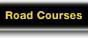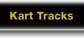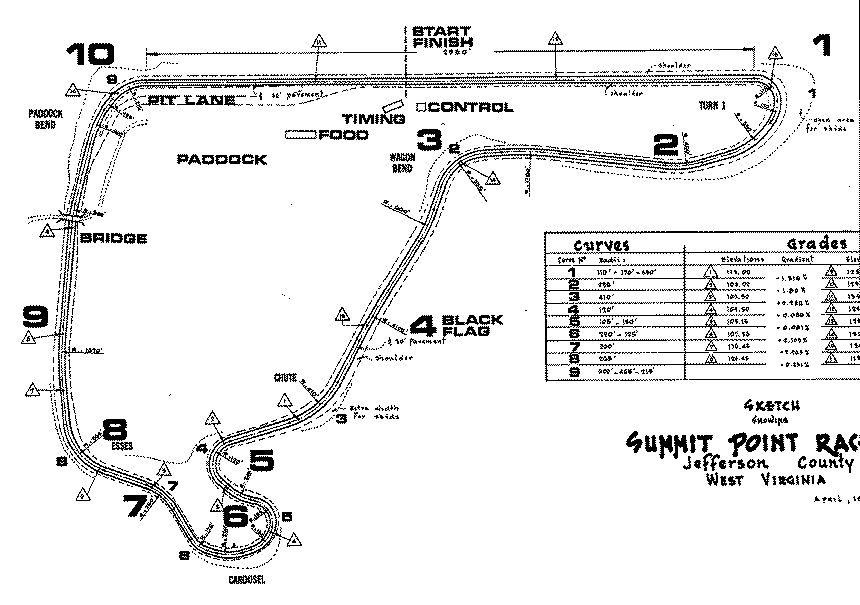## Summit Point engineering sketch

This map was supplied by Bob Hines (hinesr@csa.delta1.org).

The curve numbers in the table next to the map correspond to the small turn numbers on the map; they do not correspond to the turn numbering which is presently used. The large numbers on the map correspond to the usual flagging stations. The numbers in triangles are references for the gradient & elevation data.The radii for turn 1 (curve number 1) are (in order): 110', 170', 550'
The radius of turn 2 (next to flagging 2) is 460'
The radius of the unnumbered king betweens turns 2 and 3 is 1780'
The radius of turn 3 (curve number 2) is 235'
The radius of the first unnumbered kink after turn 3 is 660'
The radius of the second unnumbered kink after turn 3 is 4100'
The radius of turn 4 (curve number 3) is 410'
The radius of turn 5 (curve number 4) is 120'
The radius of the kink between 5 and 6a is 245'
The radii for turn 6a (curve number 5) are 105', 150'
The radius of turn 6b (curve number 6) is 220', 125'
The radius for turn 7 (curve number 7) is 260'
The radius for turn 8 (curve number 8) is 265'
The radius for the unnumbered kink between 8 and 9 is 1670'
The radius for turn 9 (the kink at the bridge) is 300'
The radii for turn 10 (curve number 9) are 600', 465', 215'.

North American Motorsports Pages / NA Websites / webmaster@na-motorsports.com